# Ratio

Unfold number 1963 in the ratio 9:2:2.

x =  1359
y =  302
z =  302

### Step-by-step explanation:

$y=2k=2\cdot 151=302$
$z=2k=2\cdot 151=302$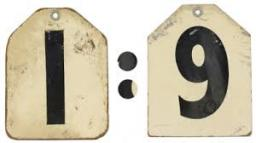Did you find an error or inaccuracy? Feel free to write us. Thank you!Tips to related online calculators
Check out our ratio calculator.

## Related math problems and questions:

• NumbersDetermine the number of all positive integers less than 4183444 if each is divisible by 29, 7, 17. What is its sum?
• Division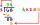Divide by the number 0.2 is the same as multiply by what number?
• Number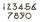What number do I think? The third is 6, and a half is 2 more than its quarter.
• Unknown numberSamuel wrote unknown number. Then he had add 200000 to the number and the result multiply by three. When it calculated he was surprised, because the result would have received anyway, if write digit to the end of original number. Find unknown number.
• Numbers divisionWith what number should be divided mixed number 2 3/4 to get 11/12?
• Unknown xIf we add to unknown number 21, then divide by 6 and then subtract 51, we get back an unknown number. What is this unknown number?
• Number ratio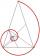Calculate two positive numbers that its ratio is 6:6 and difference was 0.
• Unknown number4/5 of a number is 276. what is 2/3 of the same number?
• Hens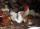11 hens will eat spilled grain from 6AM to 16 PM. At 11 hour grandmother brought 4 hens from the neighbors. At what time was grain out?
• Guess numberWith what number should be divided, number 9727 to be reduced by 70%?
• The ratio 4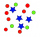The ratio of two number is 5:4 if 40% of the first number is 12, what will be 50% of the second number?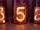What will be hexadecimal number 303 as decimal number?The root of the equation (x-19)2 -10 = x2 -11x is (equal or greater or less than zero)? ...Divide the number 72 in the ratio 7: 2 and calculate the ratio of the numbers found in this order and write down as decimal.6 numbers are in the ratio 1:5:1:5:5:5. Their sum is 242. What are the numbers?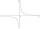Daniel thinks an integer. When he change this number at a ratio of 2:5, he got the number 2.8. Determine what number think Daniel.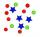Reduce the number 16 in proportion 3:2 5:4 11:8